How to Calculate a Slope

Yazan Hamzeh, Jeff Calareso, Elaine Chan
• Author
Yazan Hamzeh

I am an inspiring budding scientist, who currently works at a fertility unit. I graduated with BSc (honors) in Genetic Engineering from Jordan University of Science and Technology, and then pursued an MSc in Clinical Embryology at the University of Oxford where I graduated with merit. During the course of academic endeavors, I found a passion in writing, whether it being scientific writing or blog writing. Therefore, I am so glad to be a part of Study.com! I am looking forward to hopefully inspire the many budding students out there.

• Instructor
Jeff Calareso

Jeff teaches high school English, math and other subjects. He has a master's degree in writing and literature.

• Expert Contributor
Elaine Chan

Dr. Chan has taught computer and college level physics, chemistry, and math for over eight years. Dr. Chan has a Ph.D. in Chemistry from U. C. Berkeley, an M.S. Physics plus 19 graduate Applied Math credits from UW, and an A.B. with honors from U.C .Berkeley in Physics.

Learn how to determine the slope of a line with two points, how the slope formula is related to rise over run, and review examples of how to find the slope. Updated: 07/18/2021

Show

What is Slope?

The slope, also referred to as the gradient in mathematics, is a value given to the steepness of a line. Within two connecting points, the slope measures the steepness and direction of the line. Denoted as m, the value of the slope is directly proportional to the steepness. In other words, as the value of the slope increases, the steepness increases.

To find the slope of a line with two points, the coordinates of any two points passing through the line must be identified. Using these coordinates, the amount of change of the y-coordinates must be divided by the amount of change of x-coordinates, resulting in the slope or m. The value of a slope is not only taken into consideration in mathematics, but it can also be applied in real life. For instance, slopes can be applied by civil engineers while building roads.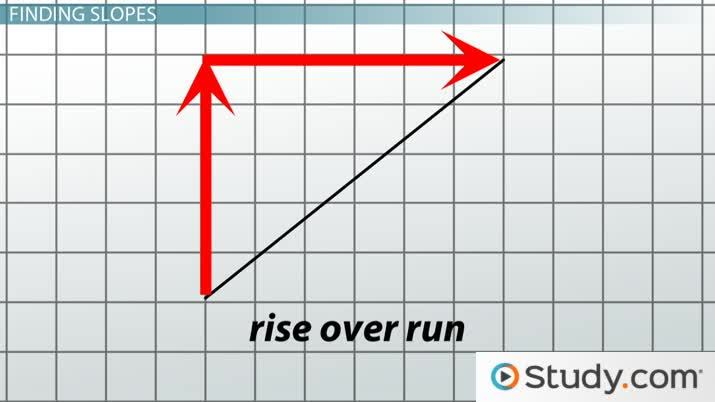An error occurred trying to load this video.

Try refreshing the page, or contact customer support.

Coming up next: How to Find and Apply the Intercepts of a Line

You're on a roll. Keep up the good work!

Replay
Your next lesson will play in 10 seconds
• 1:03 Finding Slopes
• 2:07 Types of Slopes
• 3:39 Practice Using Points
• 5:40 Slopes from Equations
• 6:59 Practice Using Equations
• 8:37 Lesson Summary
Save Save

Want to watch this again later?

Timeline
Autoplay
Autoplay
Speed Speed

The Slope Formula and Rise Over Run

Rise Over Run

The slope of a line may also be defined as rise over run or vertical change over horizontal change. As shown in the figure below, in relation to the slope formula, the rise is associated with the change of the y-coordinates while the run is associated with the change of the x-coordinates. The slope can be found by identifying the rise, or vertical change, and dividing it by the obtained run, or the horizontal change.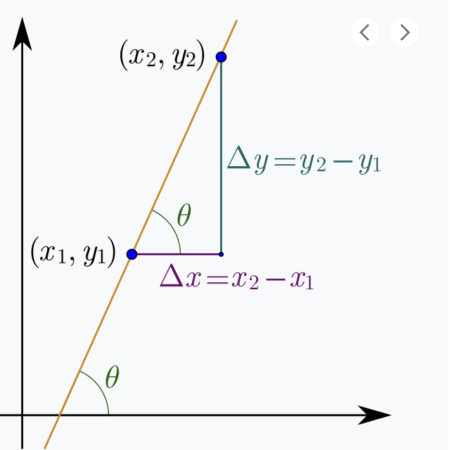Slope Formula

The slope formula can be used to obtain the slope of a line through the following steps:

1. Identify any two points on the line.
2. According to the slope formula, denote the first point as (x1, y1)
3. Denote the second point as (x2, y2).
4. Substitute the newly denoted coordinates into the formula to obtain the slope or m.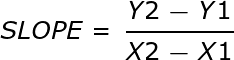In relation to the rise over run formula, the change of the y-coordinates can be defined as the rise while the change of the x-coordinates can be defined as the run. The two approaches involve dividing the vertical factors over the horizontal factors to obtain the slope. Additionally, both approaches allow the usage of any two points within the given line to find the slope.

Figuring Out Types of Slopes

Depending on the two points used to make a line, the slope can have several types:

A positive slope is represented by an increasing line that is rising upwards from left to right.

Example: A line with the two following points (2,2) and ( -1, -2) has a positive slope of {eq}\frac{4}{3}{/eq}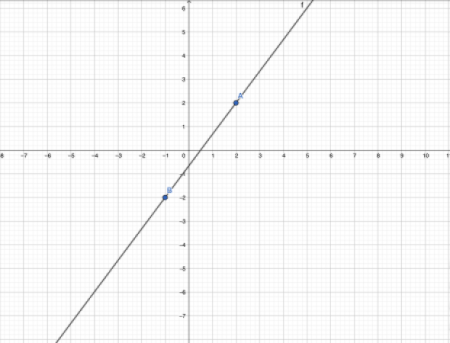A negative slope is represented by a decreasing line that is declining downwards from left to right.

Example: A line with the two following points (0,6) and (3, 3) has a negative slope of -1.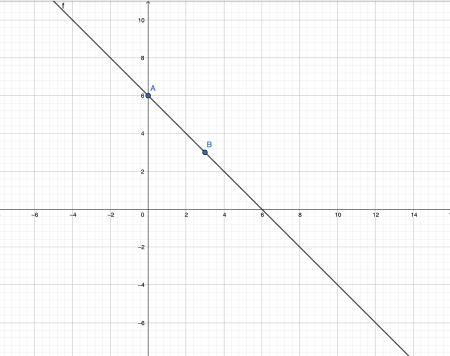A slope of zero is represented by a horizontal and constant line.

Example: A line with the two following points (-1,5) and (1, 5) has a slope of zero.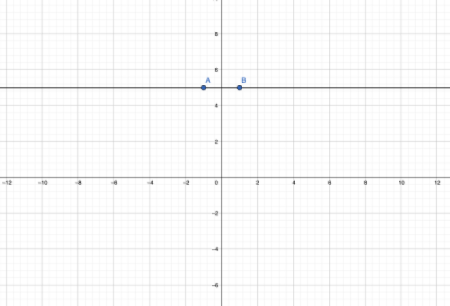An unidentified slope is represented by a vertical line.

Example: A line with the two following points (2,3) and (2, 1) has an undefined slope.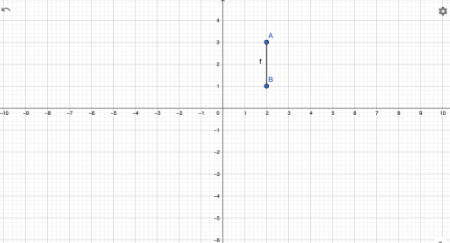How to Find Slope With Two Points

The slope of a line can be determined using the slope or rise over run formula using any two points identified on that line. Let's look at a couple of examples.

Example 1: Suppose a line contains the two following points (8,4) and (-3,7). What is the slope of the line?

1. Identify the two points on the line as (8,4) and (-3,7).
2. According to the slope formula, denote the first point (8,4) as (x1, y1).
3. Denote the second point (-3,7) as (x2, y2).
4. Substitute the newly denoted coordinates into the formula to obtain the slope or m.
• Final answer: m = {eq}\frac{4-7}{8-(-3)}{/eq} = {eq}\frac{-3}{11}{/eq}

Example 2: Suppose a line contains the two following points (1,9) and (2,12). What is the slope of the line?

1. Identify the two points on the line as (1,9) and (2,12).
2. According to the slope formula, denote the first point (1,9) as (x1, y1).
3. Denote the second point (2,12) as (x2, y2).
4. Substitute the newly denoted coordinates into the formula to obtain the slope or m.
• Final answer: m ={eq}\frac{12-9}{2-1}{/eq} = 3

To unlock this lesson you must be a Study.com Member.

Slope Problems

Problem 1:

Find an equation of the line through the point (5,2) parallel to the line 4x +6y +5 = 0

Problem 2:

Show that the lines 2x + 3y = 1 and 6x -4y -1 = 0 are perpendicular.

Problem 3:

a) Express temperature T as a function of height h, assuming a linear relationship. At ground level, T=20 degrees Celsius. At h = 1 km., T = 10 degrees Celsius.

b) What is the temperature at 2.5 km ?

Rewrite the equation as y = -2x/3 -5/6.

This equation is now in the form of the slope intercept formula ( y = mx + b ). m = -2/3.

Parallel lines have the same slope. The equation in point-slope form is

y-2= (-2/3)(x-5). We can rewrite the equation as

2x + 3y = 16.

Rewrite these equations in slope-intercept form:

y= -2x/3 + 1/3 and y = 3x/2 - 1/4

The slopes are -2/3 and 3/2.

The product of the two slopes is -1,

which is what is required for two lines to be perpendicular.

a) T = mh + b is the slope intercept form.

20 = m(0) + b; The intercept b is 20.

10 = m(1) + 20; The slope is -10. The equation is T = -10 h + 20.

b)

At h = 2.5 km, T = -10(2.5) + 20 = -5 degrees Celsius.

Register to view this lesson

Are you a student or a teacher?